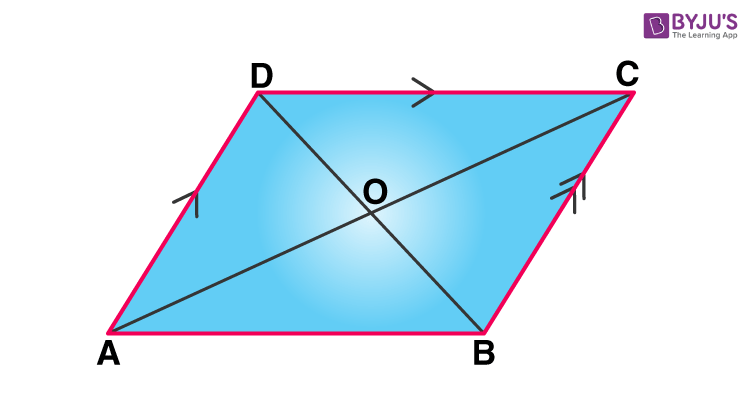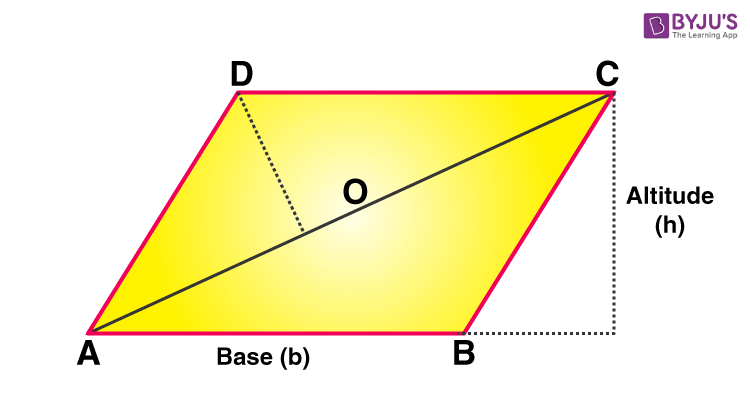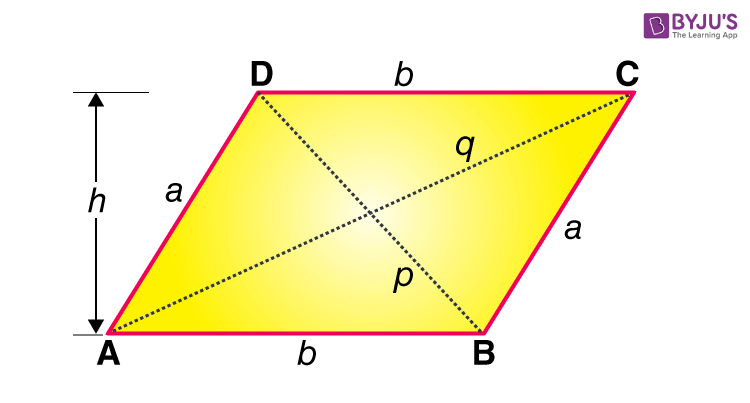# Area of Parallelogram

Area of a parallelogram is a region covered by a parallelogram in a two-dimensional plane. In Geometry, a parallelogram is a two-dimensional figure with four sides. It is a special case of the quadrilateral, where opposite sides are equal and parallel. The area of a parallelogram is the space enclosed within its four sides. Area is equal to the product of length and height of the parallelogram.

The sum of the interior angles in a quadrilateral is 360 degrees. A parallelogram has two pairs of parallel sides with equal measures. Since it is a two-dimensional figure, it has an area and perimeter. In this article, let us discuss the area of a parallelogram with its formula, derivations, and more solved problems in detail.

Also check: Mathematics Solutions

## What is the Area of Parallelogram?

The area of a parallelogram is the region bounded by the parallelogram in a given two-dimension space. To recall, a parallelogram is a special type of quadrilateral which has four sides and the pair of opposite sides are parallel. In a parallelogram, the opposite sides are of equal length and opposite angles are of equal measures. Since the rectangle and the parallelogram have similar properties, the area of the rectangle is equal to the area of a parallelogram.## Area of Parallelogram Formula

To find the area of the parallelogram, multiply the base of the perpendicular by its height. It should be noted that the base and the height of the parallelogram are perpendicular to each other, whereas the lateral side of the parallelogram is not perpendicular to the base. Thus, a dotted line is drawn to represent the height.Therefore,

 Area = b × h Square units

Where “b” is the base and “h” is the height of the parallelogram.

Let us learn the derivation of area of a parallelogram, in the next section.

## How to Calculate the Area of Parallelogram?

The parallelogram area can be calculated, using its base and height. Apart from it, the area of a parallelogram can also be evaluated, if its two diagonals are known along with any of their intersecting angles, or if the length of the parallel sides is known, along with any of the angles between the sides. Hence, there are three method to derive the area of parallelogram:

• When base and height of parallelogram are given
• When height is not given
• When diagonals are given### Area of Parallelogram Using Sides

Suppose a and b are the set of parallel sides of a parallelogram and h is the height, then based on the length of sides and height of it, the formula for its area is given by:

Area = Base × Height

A = b × h     [sq.unit]

Example: If the base of a parallelogram is equal to 5 cm and the height is 3 cm, then find its area.

Solution: Given, length of base=5 cm and height = 3 cm

As per the formula, Area = 5 × 3 = 15 sq.cm

### Area of Parallelogram Without Height

If the height of the parallelogram is unknown to us, then we can use the trigonometry concept here to find its area.

Area = ab sin (x)

Where a and b are the length of parallel sides and x is the angle between the sides of the parallelogram.

Example: The angle between any two sides of a parallelogram is 90 degrees. If the length of the two parallel sides is 3 cm and 4 cm respectively, then find the area.

Solution: Let a = 3 cm and b=4 cm

x = 90 degrees

Area = ab sin (x)

A = 3 × 4 sin (90)

A = 12 sin 90

A = 12 × 1 = 12 sq.cm.

Note: If the angle between the sides of a parallelogram is 90 degrees, then it is a rectangle.

### Area of Parallelogram Using Diagonals

The area of any parallelogram can also be calculated using its diagonal lengths. As we know, there are two diagonals for a parallelogram, which intersects each other. Suppose, the diagonals intersect each other at an angle y, then the area of the parallelogram is given by:

Area = ½ × d1 × d2 sin (y)

Check the table below to get summarised formulas of an area of a parallelogram.

All Formulas to Calculate Area of a Parallelogram
Using Base and Height A = b × h
Using Trigonometry A = ab sin (x)
Using Diagonals A = ½ × d1 × d2 sin (y)

Where,

• b = base of the parallelogram (AB)
• h = height of the parallelogram
• a = side of the parallelogram (AD)
• x = any angle between the sides of the parallelogram (∠DAB or ∠ADC)
• d1 = diagonal of the parallelogram (p)
• d2 = diagonal of the parallelogram (q)
• y = any angle between at the intersection point of the diagonals (∠DOA or ∠DOC)

Note: In the above figure,

• DC = AB = b
• AD = BC = a
• ∠DAB = ∠DCB
• O is the intersecting point of the diagonals
• ∠DOA = ∠COB
• ∠DOC = ∠AOB

## Solved Examples on Area of Parallelogram

Question 1: Find the area of the parallelogram with the base of 4 cm and height of 5 cm.

Solution:

Given:

Base, b = 4 cm

h = 5 cm

We know that,

Area of Parallelogram = b×h Square units

= 4 × 5 = 20 sq.cm

Therefore, the area of a parallelogram = 20 cm2

Question 2: Find the area of a parallelogram whose breadth is 8 cm and height is 11 cm.

Solution:

Given,

b = 8 cm

h = 11 cm

Area of a parallelogram

= b × h

= 8 × 11 cm2

= 88 cm2

Question 3: The base of the parallelogram is thrice its height. If the area is 192 cm2, find the base and height.

Solution:

Let the height of the parallelogram = h cm

then, the base of the parallelogram = 3h cm

Area of the parallelogram = 192 cm2

Area of parallelogram = base × height

Therefore, 192 = 3h × h

⇒ 3 × h2 = 192

⇒ h2 = 64

⇒ h = 8 cm

Hence, the height of the parallelogram is 8 cm, and breadth is

3 × h

= 3 × 8

= 24 cm

## Word Problem on Area of Parallelogram

Question: The area of a parallelogram is 500 sq.cm. Its height is twice its base. Find the height and base.

Solution:

Given, area = 500 sq.cm.

Height = Twice of base

h = 2b

By the formula, we know,

Area = b x h

500 = b x 2b

2b2 = 500

b2 = 250

b = 15.8 cm

Hence, height = 2 x b = 31.6 cm

## Practise Questions on Area of a Parallelogram

1. Find the area of a parallelogram whose base is 8 cm and height is 4 cm.
2. Find the area of a parallelogram with a base equal to 7 inches and height is 9 inches.
3. The base of the parallelogram is thrice its height. If the area is 147 sq.units, then what is the value of its base and height?
4. A parallelogram has sides equal to 10m and 8m. If the distance between the shortest sides is 5m, then find the distance between the longest sides of the parallelogram. (Hint: First find the area of parallelogram using distance between shortest sides)

### What is a Parallelogram?

A parallelogram is a geometrical figure that has four sides formed by two pairs of parallel lines. In a parallelogram, the opposite sides are equal in length, and opposite angles are equal in measure.

### What is the Area of a Parallelogram?

The area of any parallelogram can be calculated using the following formula:

Area = base × height

It should be noted that the base and height of a parallelogram must be perpendicular.

### What is the Perimeter of a Parallelogram?

To find the perimeter of a parallelogram, add all the sides together. The following formula gives the perimeter of any parallelogram:

Perimeter = 2 (a + b)

### What is the Area of a Parallelogram whose height is 5 cm and base is 4 cm?

The area of a perpendicular with height 5 cm and base 4 cm will be;

A = b × h

Or, A = 4 × 5 = 20 cm2

Test your Knowledge on Area of Parallelogram